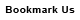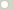# dyne meter (Torque, Metric)

## 1 dyne meter is equal to:Metric newton meter 1e-05 newton centimeter 0.001 dyne centimeter 100 kilogram-force meter 1.0197162e-06 kilogram-force centimeter 0.00010197162 gram-force meter 0.0010197162 gram-force centimeter 0.10197162 British and U.S. long ton-force foot 3.2926882e-09 short ton-force foot 3.6878107e-09 pound-force foot 7.3756215e-06 pound-force inch 8.8507458e-05 ounce-force inch 0.0014161193ConversionsAreaCapacity and VolumeCircular measureComputer storageCurrencyDistance and LengthEnergy and WorkFuel ConsumptionMass and WeightPowerPressureSpeedTemperatureTimeTorqueSearchLink to us